## TRIGONOMETRY WORD PROBLEMS WORKSHEET WITH ANSWERS

1. The angle of elevation of the top of the building at a distance of 50 m from its foot on a horizontal plane is found to be 60 °. Find the height of the building.

2.  A ladder placed against a wall such that it reaches the top of the wall of height 6 m and the ladder is inclined at an angle of 60°. Find how far the ladder is from the foot of the wall.

3.  A string of a kite is 100 meters long and t he inclination of the string  with the ground is  60°. Find the height of the kite, assuming that there is no slack in the string.

4.  From the top of the tower 30 m height a man is observing the base of a tree at an angle of depression measuring 30 ° . Find the distance between the tree and the tower.

5.  A man wants to determine the height of a light house. He measured the angle at A and found that tan A = 3/4. What is the height of the light house if A is 40 m from the base?

6. A ladder is leaning against a vertical wall makes an angle of 20° with the ground. The foot of the ladder is 3 m from the wall. Find the length of ladder.

7. A kite is flying at a height of 65 m attached to a string. If the inclination of the string with the ground is 31°,  find the length of string.

8. The length of a string between a kite and a point on the ground is 90 m. If the string makes an angle θ with the ground level such that tan θ = 15/8, how high will the kite be ?

9.  An airplane is observed to be approaching a point that is at a distance of 12 km from the point of observation and makes an angle of elevation of 50 °. Find the height of the airplane above the ground.

10.  A balloon is connected to a meteorological station by a cable of length 200 m inclined at 60 °  angle with the ground. Find the  height of the balloon from the ground (Imagine that there is no slack in the cable).Draw a sketch.Here, AB represents height of the building, BC represents distance of the building from the point of observation.

In the right triangle ABC, the side which is opposite to the angle 60° is known as opposite side (AB), the side which is opposite to 90° is called hypotenuse side (AC) and the remaining side is called adjacent side (BC).

Now we need to find the length of the side AB.

tan60° = AB/BC

√3 x 50 = AB

Approximate value of √3 is 1.732

AB = 50 (1.732)

AB = 86.6 m

So, the height of the building is 86.6 m.Here AB represents height of the wall, BC represents the distance between the wall and the foot of the ladder and AC represents the length of the ladder.

In the right triangle ABC, the side which is opposite to angle 60° is known as opposite side (AB), the side which is opposite to 90° is called hypotenuse side (AC) and remaining side is called adjacent side (BC).

Now, we need to find the distance between foot of the ladder and the wall. That is, we have to find the length of BC.

BC = (6/√3) x (√3/√3)

BC = (6√3)/3

Approximate value of √3 is 1.732.

BC = 2 (1.732)

BC = 3.464 m

So, the distance between foot of the ladder and the wall is 3.464 m.Here AB represents height of kite from the ground, BC represents the distance of kite from the point of observation.

In the right triangle ABC the side which is opposite to angle 60° is known as opposite side (AB), the side which is opposite to 90° is called hypotenuse side (AC) and remaining side is called adjacent side (BC).

Now we need to find the height of the side AB.

sinθ = opposite side/hypotenuse side

sinθ = AB/AC

sin60° = AB/100

√3/2 = AB/100

(√3/2) x 100 = AB

AB = 50√3 m

So, the height of kite from the ground 50√3 m.Here AB represents height of the tower, BC represents the distance between foot of the tower and the foot of the tree.

Now we need to find the distance between foot of the tower and the foot of the tree (BC).

tan30° = AB/BC

1/√3 = 30/BC

BC = 30(1.732)

BC = 51.96 m

So, the distance between the tree and the tower is 51.96 m.Here BC represents height of the light house, AB represents the distance between the light house from the point of observation.

In the right triangle ABC the side which is opposite to the angle A is known as opposite side (BC), the side which is opposite to 90° is called hypotenuse side (AC) and remaining side is called adjacent side (AB).

Now we need to find the height of the light house (BC).

tanA = BC/AB

Given : tanA = 3/4.

3/4 = BC/40

Multiply each side by 40.

So, the height of the light house is 30 m.Here AB represents height of the wall, BC represents the distance of the wall from the foot of the ladder.

In the right triangle ABC, the side which is opposite to the angle 20° is known as opposite side (AB),the side which is opposite to 90° is called hypotenuse side (AC) and remaining side is called adjacent side (BC).

Now we need to find the length of the ladder (AC).

Cosθ = BC/AC

Cos 20° = 3/AC

0.9397 = 3/AC

AC = 3/0.9396Here AB represents height of the kite. In the right triangle ABC the side which is opposite to angle 31° is known as opposite side (AB), the side which is opposite to 90° is called hypotenuse side (AC) and the remaining side is called adjacent side (BC).

Now we need to find the length of the string AC.

sin31° = AB/AC

0.5150 = 65/AC

AC = 65/0.5150

AC = 126.2 m

Hence, the length of the string is 126.2 m.Here AB represents height of the balloon from the ground. In the right triangle ABC the side which is opposite to angle θ is known as opposite side (AB), the side which is opposite to 90° is called hypotenuse side (AC) and remaining side is called adjacent side (BC).

tanθ = 15/8 ----> cotθ = 8/15

cscθ = √(1+ cot 2 θ)

cscθ = √(1 + 64/225)

cscθ = √(225 + 64)/225

cscθ = √289/225

cscθ = 17/15 ----> sinθ = 15/17

But, sinθ = opposite side/hypotenuse side = AB/AC.

AB/AC = 15/17

AB/90 = 15/17

So, the height of the tower is 79.41 m.Here AB represents height of the airplane from the ground. In the right triangle ABC the side which is opposite to angle 50° is known as opposite side (AB), the side which is opposite to 90° is called hypotenuse side (AC) and remaining side is called adjacent side (BC).

From the figure given above, AB stands for the height of the airplane above the ground.

sin50° = AB/AC

0.7660 = h/12

0.7660 x 12 = h

So, the height of the airplane above the ground is 9.192 km.Here AB represents height of the balloon from the ground. In the right triangle ABC the side which is opposite to angle 60° is known as opposite side (AB), the side which is opposite to 90° is called hypotenuse (AC) and the remaining side is called as adjacent side (BC).

From the figure given above, AB stands for the height of the balloon above the ground.

sin60° = AB/200

√3/2 = AB/200

AB = (√3/2) x 200

AB = 100(1.732)

AB = 173.2 m

So, the height of the balloon from the ground is 173.2 m.

Kindly mail your feedback to   [email protected]

• Sat Math Practice
• SAT Math Worksheets
• PEMDAS Rule
• BODMAS rule
• GEMDAS Order of Operations
• Math Calculators
• Transformations of Functions
• Order of rotational symmetry
• Lines of symmetry
• Compound Angles
• Quantitative Aptitude Tricks
• Trigonometric ratio table
• Word Problems
• Times Table Shortcuts
• 10th CBSE solution
• PSAT Math Preparation
• Laws of Exponents## Recent Articles## Like and Unlike Fractions Worksheets

Nov 20, 23 03:23 AM

## Like and Unlike Fractions Definition

Nov 20, 23 03:06 AM## Printable Math Worksheets

Nov 20, 23 02:17 AM• English Language Arts
• Graphic Organizers
• Social Studies
• Teacher Printables
• Foreign Language

Home > Math Worksheets > Word Problems > Trigonometry

## These types of problems focus on the lengths of sides and angles within a triangle. After all that is what trigonometry is all about. Start to approach these problems by focusing on understanding the figures that are involved and model this by drawing diagrams. On the diagram highlight all the information that we know about each stage of the diagram. Make sure that if a unit of measure is attached to that information that you show that as well. We then start to analyze the system and identify if Pythagorean Theorem comes into play or if we can apply various trigonometric functions to determine the angle of elevation or depression.

In these worksheets, your students will solve word problems involving trigonometry. Diagrams are included to accompany some problems. These are moderately complex problems and a sound understanding of trigonometry is required in order for students to be successful with these worksheets. There are six worksheets in this set. This set of worksheets contains lessons, step-by-step solutions to sample problems, and both simple and more complex problems. It also includes ample worksheets for students to practice independently. Students may require extra paper on which to do their calculations. Most worksheets contain between eight and eleven problems. When finished with this set of worksheets, students will be able to solve word problems that involve trigonometry. These worksheets explain how to solve word problems by using trigonometry. Sample problems are solved and practice problems are provided.

## Get Free Worksheets In Your Inbox!

Trigonometry word problems worksheets, click the buttons to print each worksheet and answer key., trigonometric word problem lesson.

This worksheet explains how to solve word problems by using trigonometry. A sample problem is solved, and two practice problems are provided.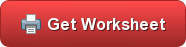You are stationed at a radar base and you observe an unidentified plane at an altitude h = 2000 m flying towards your radar base at an angle of elevation = 30o. After exactly one minute, your radar sweep reveals that the plane is now at an angle of elevation = 60o maintaining the same altitude. What is the speed (in m/s) of the plane?A fisherman drops a boat anchor and pays out 93 meter of anchor rope. The rope makes an angle of depression of 59.5 degrees with the horizontal. Assuming a level bottom, how deep in the water?

## Review and Practice

What is the measure of the diagonal of the rectangle ABHG ?

A tree in a back yard is to be cut down. The base of the tree from the house is 23 meters away, and the angle of elevation from the house to the top of the tree is 45.5 degrees. Could the tree hit the house when it is cut down?

An aerial 30 meter tail is to be supported by 2 guy wires each 50 meter from its base. How long will each guy wire be?

## Trigonometric Word Problems Worksheets

How to Solve Trigonometric Word Problems - Students often get to solving the Trigonometry Problems stage when they are in the ninth grade, and it can get tricky at times. In this topic, we will be covering a general or basic idea regarding Solving Trigonometry Problems along with some useful tips. In mathematics, it is essential to understand how you understand something rather than memorizing the steps. Trigonometry is the study of triangles. Let’s discuss some of the tips. 1. The first step involves remembering the formulas and definitions. Unless and until you are familiar with the identities and the background information of a trigonometric problem, till then, you cannot get better at Solving Trigonometry Problems. 2. The second tip is practice. The real reason why most students struggle with solving trigonometric problems is because of a lack of practice. Learning the formulas is the easier part; the bigger challenge is to maintain the continuous practice of every single formula and learning variations of problems. 3. Practice your way into difficulty. If you are getting too comfortable with a particular level of difficulty, then it is recommended you increase the level and do more difficult ones.

## Basic Lesson

Guides students solving various trigonometry based word problems. What is formula to find x?

## Intermediate Lesson

Demonstrates how to solve complex absolute value problems. A point on the ground 125 feet from the foot of a tree, the angle of elevation of the top of the tree is 32 degrees. Find to the nearest foot, the height of the tree.

## Independent Practice 1

A really great activity for allowing students to understand the concept of trigonometric word problems. A ladder leans on a building. The foot of ladder is 6 feet from the building and reaches a height of 14 feet on the building. Find the angle the ladder makes with the ground?

## Independent Practice 2

Students find the final value of trigonometric word problems in assorted problems. The answers can be found below. Calculate the height of a tree, the angle of elevation from a point A is 70 degrees. The distance is 18 m from the base of the tree. How high is the tree?

## Homework Worksheet

Students are provided with problems to achieve the concepts of trigonometric word problems.

This tests the students ability to evaluate trigonometric word problems. Example: A kite is 33 m above the ground. The kite string makes an angle of 38 degrees with the ground. Assuming that the string is taut, how much string is out?

## Homework and Quiz Answer Key

Answers for the homework and quiz.

Answers for the lesson and practice sheets.

## Trig. Homework

Here's a great excuse to try the next time you forget your trigonometry homework: " I locked the paper in my trunk but a four-dimensional dog got in and ate it."

## HIGH SCHOOL

• ACT Tutoring
• SAT Tutoring
• PSAT Tutoring
• ASPIRE Tutoring
• SHSAT Tutoring
• STAAR Tutoring

• MCAT Tutoring
• GRE Tutoring
• LSAT Tutoring
• GMAT Tutoring
• AIMS Tutoring
• HSPT Tutoring
• ISEE Tutoring
• ISAT Tutoring
• SSAT Tutoring

## math tutoring

• Elementary Math
• Pre-Calculus
• Trigonometry

## science tutoring

Foreign languages.

• Mandarin Chinese

## elementary tutoring

• Computer Science

## Search 350+ Subjects

• Video Overview
• Tutor Selection Process
• Online Tutoring
• Mobile Tutoring
• Instant Tutoring
• How We Operate
• Our Guarantee
• Impact of Tutoring
• Reviews & Testimonials
• Media Coverage

## Trigonometry : Solving Word Problems with Trigonometry

Study concepts, example questions & explanations for trigonometry, all trigonometry resources, example questions, example question #1 : solving word problems with trigonometry.You can draw the following right triangle using the information given by the question: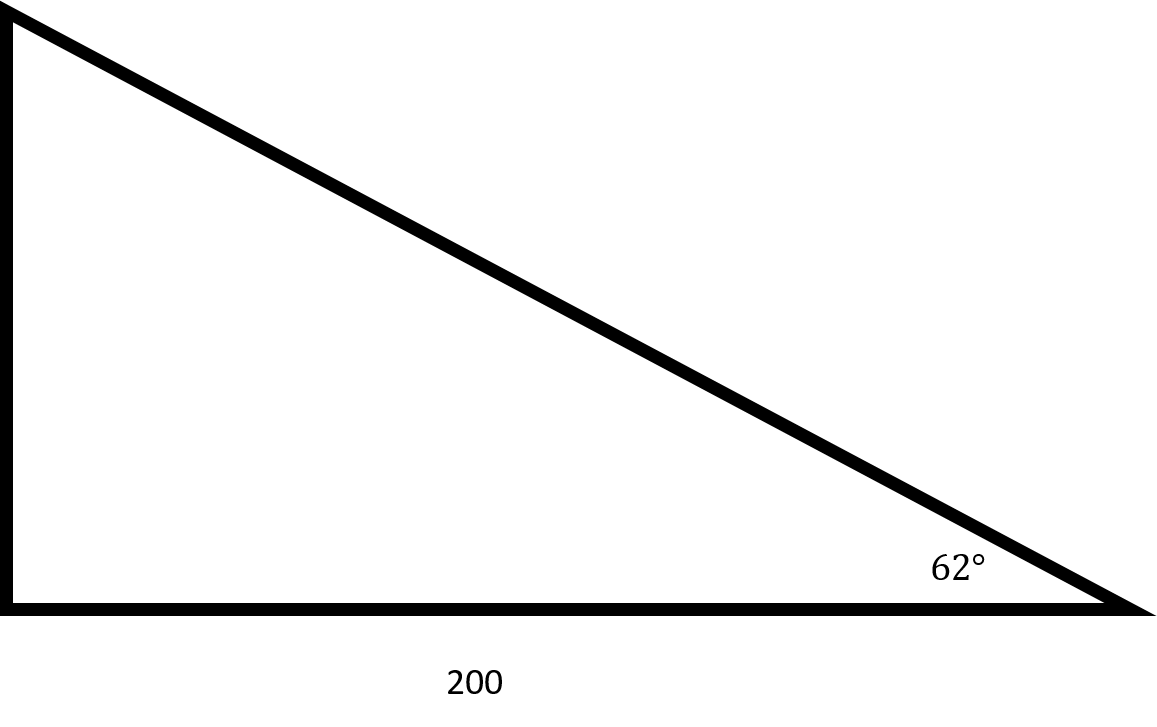Since you want to find the height of the platform, you will need to use tangent.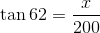You can draw the following right triangle from the information given by the question.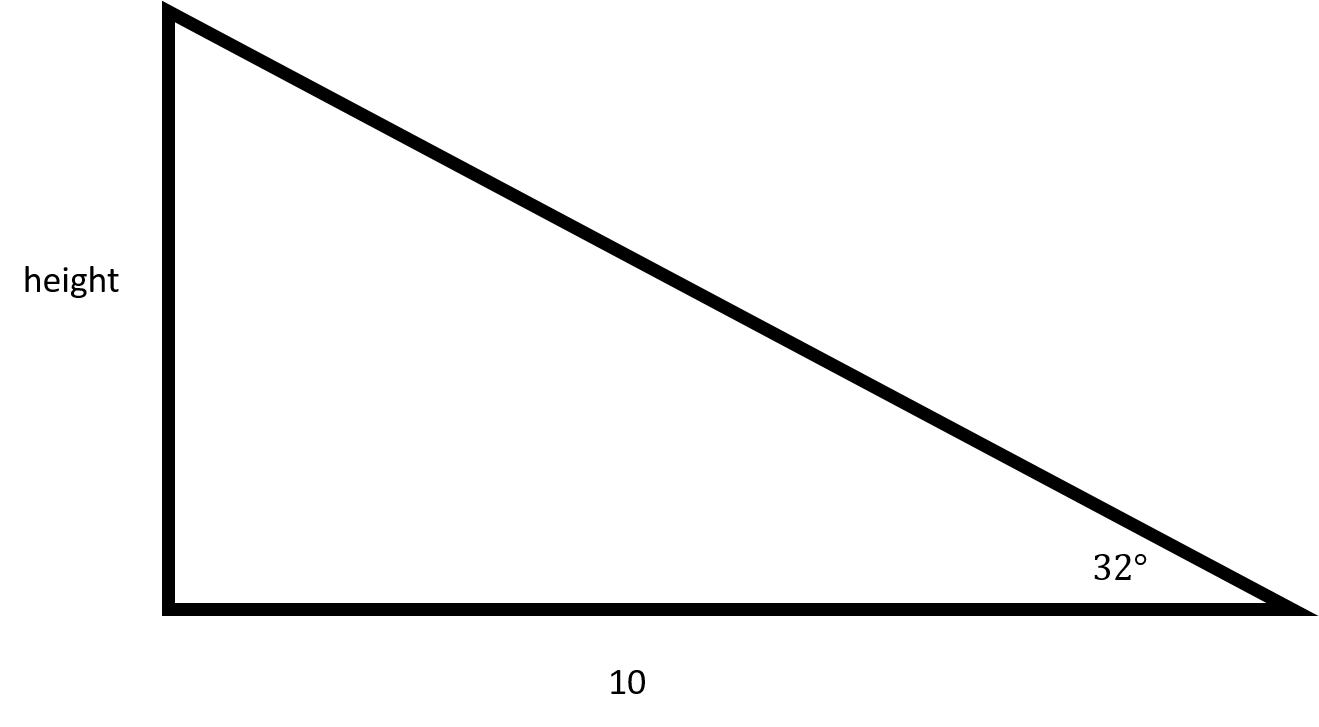In order to find the height of the flagpole, you will need to use tangent.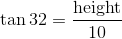You can draw the following right triangle from the information given in the question: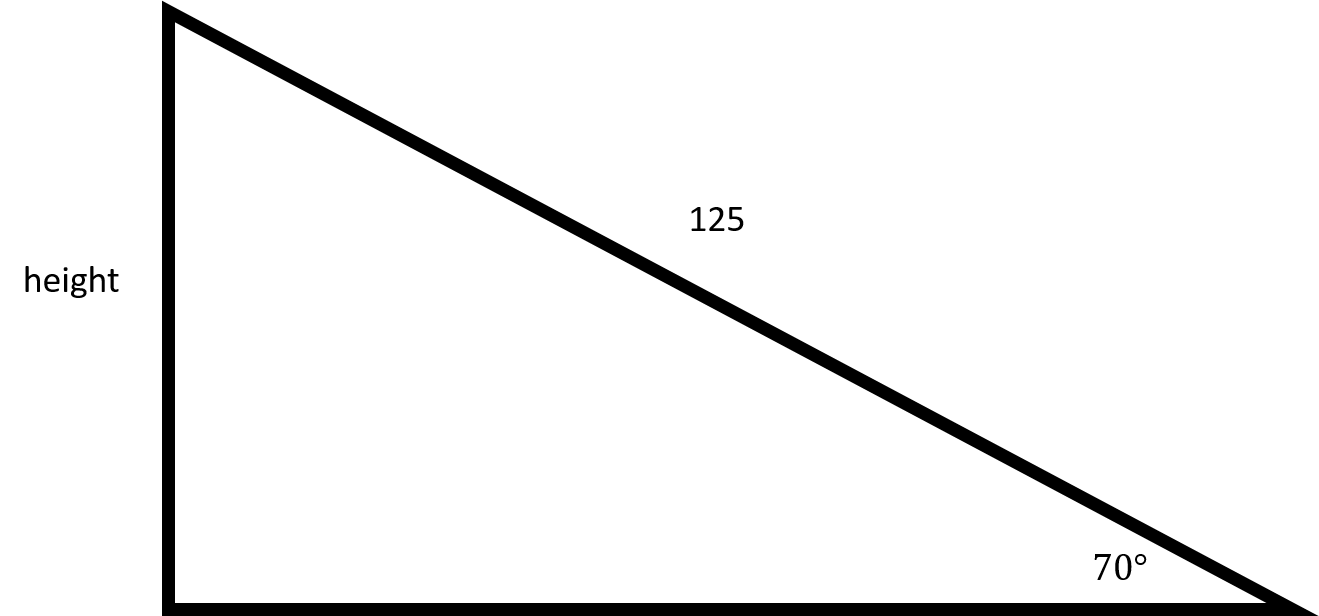In order to find out how far up the ladder goes, you will need to use sine.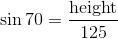## Example Question #4 : Solving Word Problems With Trigonometry

In right triangle ABC, where angle A measures 90 degrees, side AB measures 15 and side AC measures 36, what is the length of side BC?This triangle cannot exist.## Example Question #5 : Solving Word Problems With Trigonometry

A support wire is anchored 10 meters up from the base of a flagpole, and the wire makes a 25 o angle with the ground. How long is the wire, w? Round your answer to two decimal places.

23.81 meters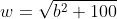28.31 meters

21.83 meters

To make sense of the problem, start by drawing a diagram. Label the angle of elevation as 25 o , the height between the ground and where the wire hits the flagpole as 10 meters, and our unknown, the length of the wire, as w.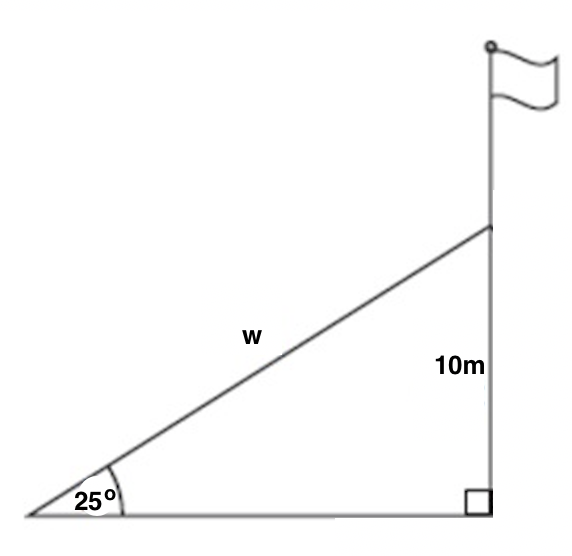Now, we just need to solve for w using the information given in the diagram. We need to ask ourselves which parts of a triangle 10 and w are relative to our known angle of 25 o . 10 is opposite this angle, and w is the hypotenuse. Now, ask yourself which trig function(s) relate opposite and hypotenuse. There are two correct options: sine and cosecant. Using sine is probably the most common, but both options are detailed below.

We know that sine of a given angle is equal to the opposite divided by the hypotenuse, and cosecant of an angle is equal to the hypotenuse divided by the opposite (just the reciprocal of the sine function). Therefore: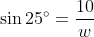To solve this problem instead using the cosecant function, we would get: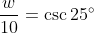The reason that we got 23.7 here and 23.81 above is due to differences in rounding in the middle of the problem.## Example Question #6 : Solving Word Problems With Trigonometry

When the sun is 22 o above the horizon, how long is the shadow cast by a building that is 60 meters high?

To solve this problem, first set up a diagram that shows all of the info given in the problem.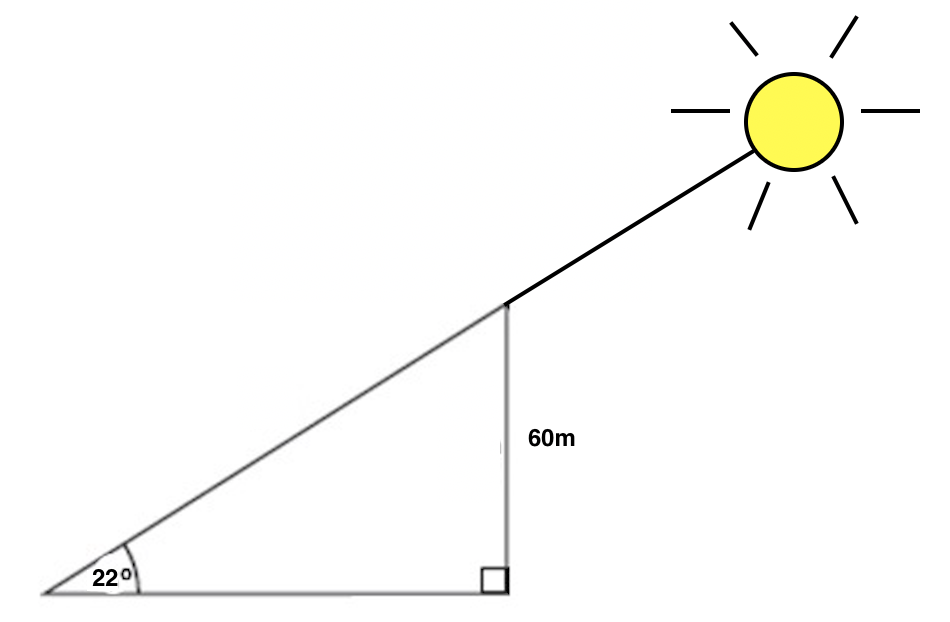Next, we need to interpret which side length corresponds to the shadow of the building, which is what the problem is asking us to find. Is it the hypotenuse, or the base of the triangle? Think about when you look at a shadow. When you see a shadow, you are seeing it on something else, like the ground, the sidewalk, or another object. We see the shadow on the ground, which corresponds to the base of our triangle, so that is what we'll be solving for. We'll call this base b.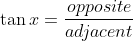Therefore the shadow cast by the building is 150 meters long.

If you got one of the incorrect answers, you may have used sine or cosine instead of tangent, or you may have used the tangent function but inverted the fraction (adjacent over opposite instead of opposite over adjacent.)

## Example Question #7 : Solving Word Problems With Trigonometry

From the top of a lighthouse that sits 105 meters above the sea, the angle of depression of a boat is 19 o . How far from the boat is the top of the lighthouse?

423.18 meters

318.18 meters

36.15 meters

110.53 meters

To solve this problem, we need to create a diagram, but in order to create that diagram, we need to understand the vocabulary that is being used in this question. The following diagram clarifies the difference between an angle of depression (an angle that looks downward; relevant to our problem) and the angle of elevation (an angle that looks upward; relevant to other problems, but not this specific one.) Imagine that the top of the blue altitude line is the top of the lighthouse, the green line labelled GroundHorizon is sea level, and point B is where the boat is.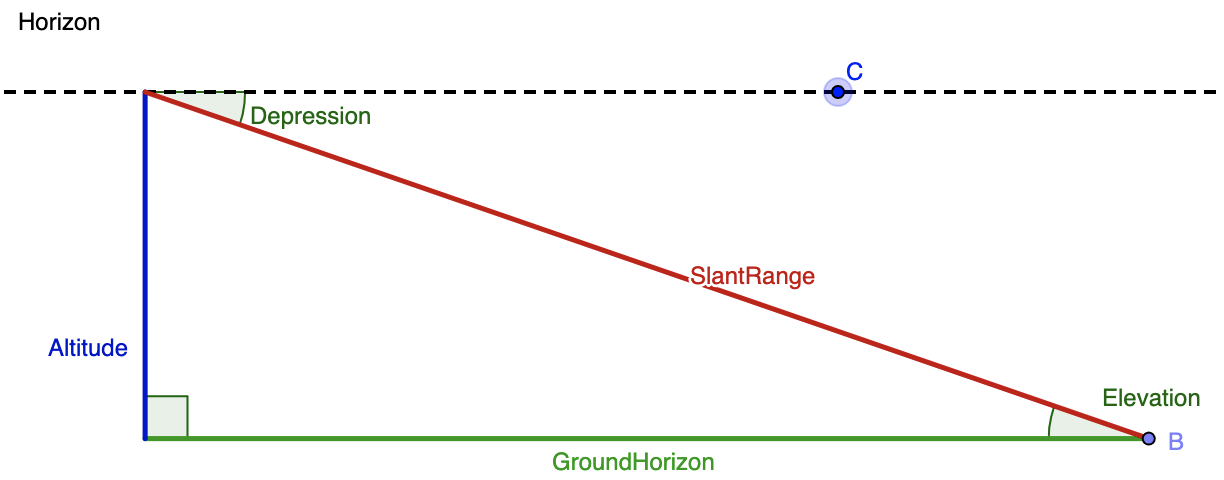Merging together the given info and this diagram, we know that the angle of depression is 19 o  and and the altitude (blue line) is 105 meters. While the blue line is drawn on the left hand side in the diagram, we can assume is it is the same as the right hand side. Next, we need to think of the trig function that relates the given angle, the given side, and the side we want to solve for. The altitude or blue line is opposite the known angle, and we want to find the distance between the boat (point B) and the top of the lighthouse. That means that we want to determine the length of the hypotenuse, or red line labelled SlantRange. The sine function relates opposite and hypotenuse, so we'll use that here. We get: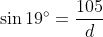## Example Question #8 : Solving Word Problems With Trigonometry

Angelina just got a new car, and she wants to ride it to the top of a mountain and visit a lookout point. If she drives 4000 meters along a road that is inclined 22 o to the horizontal, how high above her starting point is she when she arrives at the lookout?

9.37 meters

1480 meters

3708.74 meters

10677.87 meters

1616.1 meters

As with other trig problems, begin with a sketch of a diagram of the given and sought after information.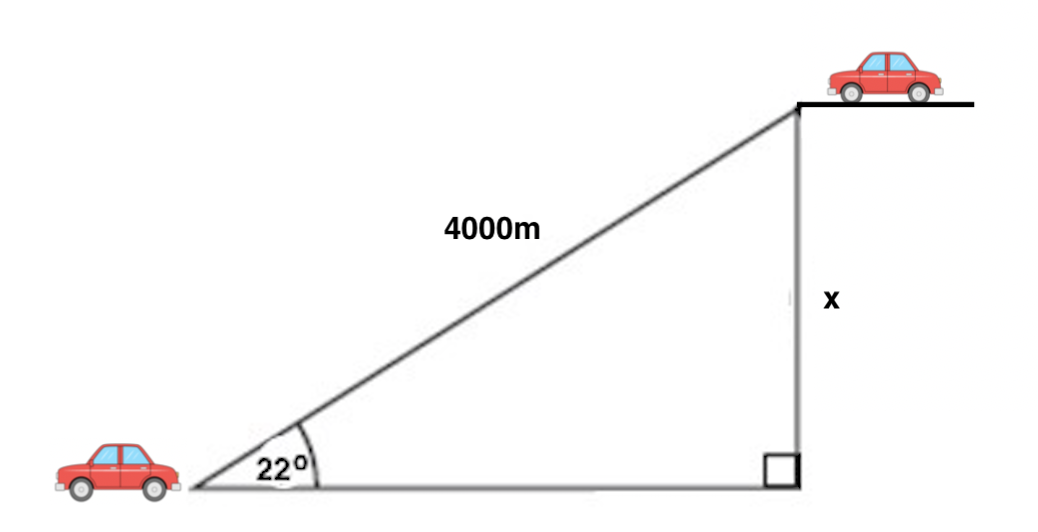Angelina and her car start at the bottom left of the diagram. The road she is driving on is the hypotenuse of our triangle, and the angle of the road relative to flat ground is 22 o . Because we want to find the change in height (also called elevation), we want to determine the difference between her ending and starting heights, which is labelled x in the diagram. Next, consider which trig function relates together an angle and the sides opposite and hypotenuse relative to it; the correct one is sine. Then, set up: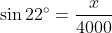Therefore the change in height between Angelina's starting and ending points is 1480 meters.

## Example Question #9 : Solving Word Problems With Trigonometry

Two buildings with flat roofs are 50 feet apart. The shorter building is 40 feet tall. From the roof of the shorter building, the angle of elevation to the edge of the taller building is 48 o . How high is the taller building?

To solve this problem, let's start by drawing a diagram of the two buildings, the distance in between them, and the angle between the tops of the two buildings. Then, label in the given lengths and angle.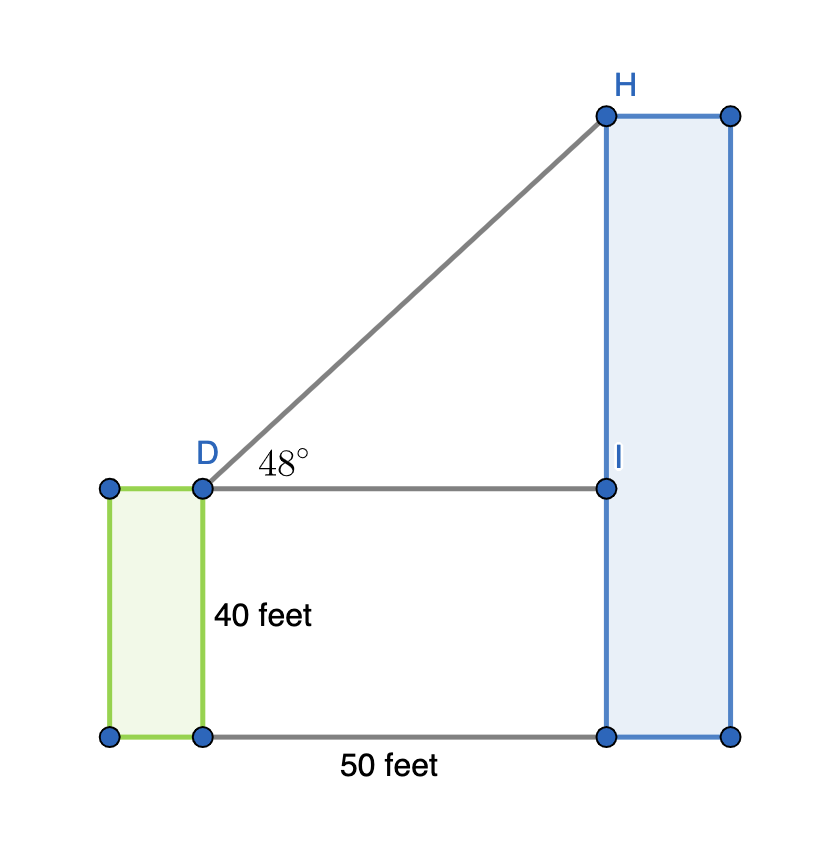## Example Question #10 : Solving Word Problems With Trigonometry

Two buildings with flat roofs are 80 feet apart. The shorter building is 55 feet tall. From the roof of the shorter building, the angle of elevation to the edge of the taller building is 32 o . How high is the taller building?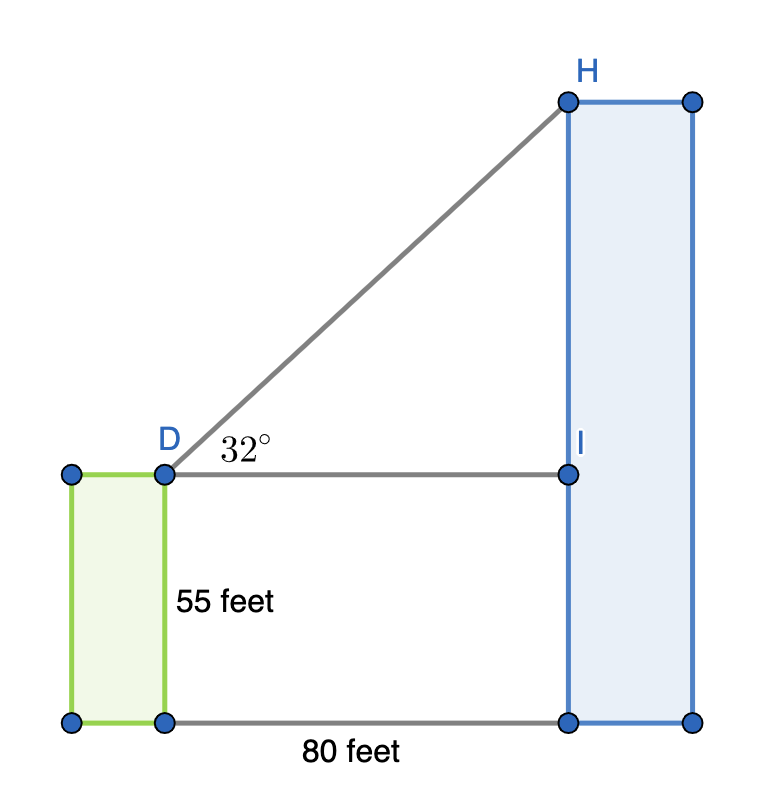## Report an issue with this question

If you've found an issue with this question, please let us know. With the help of the community we can continue to improve our educational resources.

## DMCA Complaint

If you believe that content available by means of the Website (as defined in our Terms of Service) infringes one or more of your copyrights, please notify us by providing a written notice (“Infringement Notice”) containing the information described below to the designated agent listed below. If Varsity Tutors takes action in response to an Infringement Notice, it will make a good faith attempt to contact the party that made such content available by means of the most recent email address, if any, provided by such party to Varsity Tutors.

Your Infringement Notice may be forwarded to the party that made the content available or to third parties such as ChillingEffects.org.

Please be advised that you will be liable for damages (including costs and attorneys’ fees) if you materially misrepresent that a product or activity is infringing your copyrights. Thus, if you are not sure content located on or linked-to by the Website infringes your copyright, you should consider first contacting an attorney.

You must include the following:

Send your complaint to our designated agent at:

Charles Cohn Varsity Tutors LLC 101 S. Hanley Rd, Suite 300 St. Louis, MO 63105

Or fill out the form below:

## Contact Information

Complaint details.## Find the Best Tutors## Trigonometry Worksheets

Enjoy these free sheets. Each one has model problems worked out step by step, practice problems, as well as challenge questions at the sheets end. Plus each one comes with an answer key.

(This sheet is a summative worksheet that focuses on deciding when to use the law of sines or cosines as well as on using both formulas to solve for a single triangle's side or angle)

• Law of Sines
• Ambiguous Case of the Law of Sines
• Law Of Cosines
• Sine, Cosine, Tangent, to Find Side Length
• Sine, Cosine, Tangent Chart
• Inverse Trig Functions
• Real World Applications of SOHCATOA
• Mixed Review
• Vector Worksheet
• Unit Circle Worksheet
• Graphing Sine and Cosine Worksheet

## Ultimate Math Solver (Free) Free Algebra Solver ... type anything in there!

Popular pages @ mathwarehouse.com.• US EN US English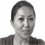• Partnerships
• Study Guide

Home » Mathematics » Pre Calculus » Word Problems and Applications of Trigonometry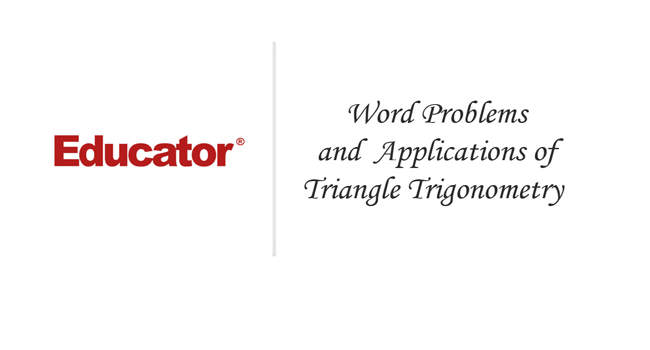Professor Murray

## Word Problems and Applications of Trigonometry

Section 8: Applications of Trigonometry: Lecture 5 | 34:25 min

In these word problems and applications of trigonometry, you'll use the knowledge of the previous lectures: The SOHCAHTOA, The Law of Sines, The Law of Cosines, and Heron's Formula. Remember that the SOHCAHTOA is used in right triangles only, while the other rules can be used in any triangle. In this lecture, you'll learn how to find the height of a telephone pole if you are given the length of its shadow and the angle that the sun's rays make with the ground. In other examples, you'll work with bridge length, roads to a town and some other real life situations.## Copy & Paste this embed code into your website’s HTML

• Show resize button - Allow users to view the embedded video in full-size.

Working on the solution...

Main formulas :

• Master formula for right triangles: SOHCAHTOA!
• The Law of Sines (in any triangle)
• The Law of Cosines (in any triangle)
• Heron's Formula (in any triangle)

Example 1 :

Example 2 :

Example 3 :

Example 4 :

Example 5 :

Lecture Slides are screen-captured images of important points in the lecture. Students can download and print out these lecture slide images to do practice problems as well as take notes while watching the lecture.• Formulas to Remember 0:11
• Law of Sines
• Law of Cosines
• Heron's Formula
• Example 1: Telephone Pole Height 4:01
• Example 2: Bridge Length 7:48
• Example 3: Area of Triangular Field 14:20
• Extra Example 1: Kite Height
• Extra Example 2: Roads to a Town

## Precalculus with Limits Online Course

Transcription: word problems and applications of trigonometry.

We are trying some more examples of applied trigonometry to solve word problems. 0000

In this first one, we are given a child flying a kite on 200ft of string and the kite’s string makes an angle of 40 degrees with the ground. 0006

We want to figure out how high is the kite of the ground. 0014

Remember, whenever you have these word problems, the important thing is to draw a picture right away. 0019

That helps you convert from lots of words into some triangles or geometric picture where you can invoke the equation that you know. 0023

Let me draw this now, the child is flying a kite on 200ft of string and the kite’s string makes an angle of 40 degrees with the ground, that is a 40 degree angle. 0034

I want to figure out how high the kite is off the ground so let me draw in something to mark the height here. 0050

There is the kite up there and here is the child, we are not going to worry about how tall the child is because that really will not make a difference. 0066

We are trying to find this distance right here, that is the height of the kite off the ground. 0077

This is a right triangle so we can use our formulas for right triangles which is SOHCAHTOA. 0086

Remember SOHCAHTOA only works for right triangles, if you do not have a right triangle then you will going use something like the law of sin or law of cos, or heron’s formula. 0092

If you have a right triangle, SOHCAHTOA is usually faster. 0102

Let us see what I know here, I do not really know anything except the hypotenuse of this triangle and one angle, and then this is the opposite side to the angle that I know. 0108

I’m going to use this part of SOHCAHTOA, sin=opposite/hypotenuse, sin(theta)=opposite/hypotenuse that is because I know the hypotenuse and I’m looking for the opposite side there. 0122

Sin(40)=opposite/200 and if I solve that by for the opposite side, I will get the opposite side as equal to (200) sin (40). 0140

Let me check on my calculator what that is, since that is not a common value, that is not one that I just remember. 0162

What I get is an approximate value of 128.6 and we are told that the unit of measurement here is feet, so, 128.6ft. 0171

That one was a pretty quick one, the reason it was quick because we really had a right angle there so we are able to use SOHCAHTOA. 0199

If we did not have a right angle and we had to use the law of cos or law of sin, it probably would have been longer. 0205

Let us recap what it took to do that problem. 0212

First of all, you read the word and try to make a sense of it then you draw a picture. 0215

It is very important that you draw a picture to illustrate what is going on. 0219

We drew a picture with a child here, the kite here, and we know that the child is flying a kite on 200ft of string. 0223

We fill that in, we are told that the kite’s string makes a 40 degree angle with the ground and we draw that in. 0233

We are trying to find how high is the kite? We draw in the height of the kite. 0240

We have a right angle, definitely a candidate for SOHCAHTOA and the reason I picked sin is because I want to find the opposite side to the angle and I know the hypotenuse. 0247

That is why I worked with sin, sin=opposite/hypotenuse. 0258

I filled in the values that I know 40 and 200, and I just solved it for the opposite. 0263

I got a number and then I checked back to see that we are using feet. 0268

That tells me that my unit for measurement for the answer should be feet. 0274

Our last example here is another pretty wordy one, two straight roads lead from different points along the coast to an in land town. 0000

Surveyors working on the coast measure that the roads are 12 miles apart and make angles of 40 degrees and 110 degrees with the coast. 0009

I want to find out how far is it to the town along each one of the roads? 0019

This is a pretty complicated one, when you get like one like this with lots of words, it is absolutely essential that the first thing you do is draw a picture. 0025

Let me draw a picture, that is supposed to be the coastline there. 0034

Those are supposed to be fish swimming in the ocean and we have got two different points along the coast. 0047

Two roads leads from these points to an in land town and these roads make angles of 40 degrees and 110 degrees with the coast. 0055

That is about 110 and that is about 40, we know that straight roads go off and they go to some town somewhere in land. 0069

The last thing we are told is that the roads are 12 miles apart, I’m going to write in my third side as 12 miles there. 0086

We want to figure out how far it is to the town along in each of the roads. 0099

First of all, let us look at this triangle we got here. 0104

What I have been given is two angles of the triangle and the side in between them, so we have been given an (angle, side, angle) situation. 0108

When you are given an (angle, side, angle) situation, the thing you want to check is whether those angles are really legitimate. 0120

In other words, whether they add up to less than 180 degrees. 0127

The angles sum up to, in this case (110+40=150) which is less than 180, which is good, there is a unique solution. 0132

I’m going to label everything I know here, I’m going to label that unknown angle as (C) and the others are (A and B). 0160

I will label my sides (a, b – remember you put the sides opposite the angles of the same letter and that side that we know is 12 is (c). 0172

Now I have labeled everything I know and I want to find the length of the roads, that is (a and b), so how can I find those? 0189

This is an (angle, side, angle) situation, (angle, side, angle) if you remember that is the one you want to solve using the law of sin. 0202

That one you want to solve with the law of sin, it does not work very well with the law of cos. 0218

Certainly, it does not work with SOHCAHTOA because we do not have a right angle here. 0223

We are going to use the law of sin, let me remind you what that is, that says sin(A)/a=sin(B)/b=sin(C)/c. 0227

That is the law of sin and we want to use that to solve for (a) and (b). 0245

Let me work on (a), I will write down sin(A)/(a)=sin(C)/c, the reason I’m going to that is because I know what (c) is and I do not know what any of the others are. 0251

I can find (C) very easily, (C) is 180-(A)-(B), that is because the three angles of a triangle add up to 180. 0271

That is 180-110-40, 180-150=30, so (C) is 30 degrees, that is not my full answer to the problem but that is going to be useful to me. 0284

Sin(A)=sin(110), sin(a)= I do not know yet, sin(C)=sin(30), sin(c)=12. 0307

I’m going to cross multiply to solve for (a), 12 sin(110)=(a)sin(30), if I solve that for (a), (a)=(12)sin (110)/sin(30). 0325

I’m going to reduce that using my calculator, remember to set your calculators on degree mode if that is what you are using. 0349

If you use radian mode, your calculator will interpret this as a 110 radians and 30 radians and will give you answers that do not make sense. 0355

I’m going to simplify (12)sin(110)/sin(30), it tells me that it is approximately 22.6. 0364

The unit of measurement here is miles be we were told here that the measurement on the coast was given as 12 miles. 0388

That tells us how long the A road is, 22.6 miles. 0398

Now let us figure out how long the B road is, again we are using the law of sin. 0405

Sin(B)/(b)=sin(C)/sin(c), I fill in what I know there. 0409

I know that (B) is 40 degrees, I do not know (b), that is what I’m solving for, sin(C) is sin(30), (c) is (12). 0421

I will cross multiply there, I get (12)sin(40)=(B)sin(30), I want to solve for (b), (b)=(12)sin(40)/sin(30). 0441

I will plug that into my calculator. 0470

Make sure that it is in degree mode and I get an approximate answer of 15.4 miles for (b). 0477

Now I have the lengths of those two roads to the town in land. 0493

Let us recap what we did there, we were graded with this long problem and lots of words. 0498

The first thing to do is draw a picture and try to identify everything you are being given in the problem. 0502

We have different points along the coast, we have an in land town, we measure the roads are 12 miles apart. 0509

I filled that 12 miles into my distance along the coast, I filled in the two angles, and that was really all the problem gave me. 0517

But that was enough to set up a triangle and to notice that I have (angle, side, angle). 0529

Once I know that I have (angle, side, angle) I can the measure of the angles, make sure it is less than 180 and that tells me that it has a unique solution. 0535

Now with (angle, side, angle) it is really not a good one to work with cos, certainly it does not work with SOHCAHTOA because we do not have a right angle. 0545

Remember that SOHCAHTOA only works with right angles. 0554

We are stuck using the law of sin, which actually works very well for (angle, side, angle). 0558

First thing we had to do is find that third angle, the way we did that was by noticing that the angles add up to 180 degrees. 0564

We used that to find the value of the third angle, that the value of angle C is 30 degrees. 0572

We were able to plug that in to these incarnations of the law of sines, sin(A)/(a)=sin(C)/(c). 0578

We plug in everything we know and the only thing missing is this (a). 0588

We were able to solve this down and get (a) equal to 22.6 and we figured out that the unit of measurement is miles. 0594

That is why we said that the answer was in term of miles. 0603

We used the law of sines again, sin(B)/(b)=sin(C)/(c) and fill in everything we know, reduced it down, solve for (b) and give our answer in terms of miles. 0607

That tells us the length of those two roads that finishes off this problem. 0620

That also finishes the lecture on word problems and applications of triangle trigonometry. 0624

Thanks for watching this trigonometry lectures on www.educator.com. 0631

Hi, these are the trigonometry lectures for educator.com, and today we're going to look at some word problems and some applications of triangle trigonometry. 0000

We're going to be using all the major formulas that we've learned in the previous lectures. 0009

I hope you remember those very well. 0014

The master formula which works for right triangles is SOH CAH TOA. 0016

You can remember that as Some Old Horse Caught Another Horse Taking Oats Away. 0021

Remember, that only works in a right triangle. 0025

If you have an angle θ, that relates the sine, cosine and tangent of θ to the hypotenuse, the side opposite θ, and the side adjacent to θ. 0030

You interpret that as the sin(θ) is equal to opposite over hypotenuse, the cos(θ) is equal to adjacent over hypotenuse, and the tan(θ) is equal to opposite over adjacent. 0043

The law of sines works in any triangle. 0056

Let me draw a generic triangle here, doesn't have to be a right triangle for the law of sines to work, so a, b, and c ... 0060

Generally, you'd label the angles with capital letters, and label the sides with lowercase letters opposite the corner with the same letter. 0071

That makes this a, this is b, and this is c. 0082

The law of sines says that sin(A)/a=sin(B)/b=sin(C)/c. 0086

That's the law of sines. 0106

Law of cosines also works in any triangle. 0108

Let me remind you what that one is. 0112

We had a whole lecture on it earlier, but just to remind you quickly, it says that c 2 =a 2 +b 2 -2abcos(C). 0114

That's useful when you know all three sides, you can figure out an angle very quickly using the law of cosines. 0129

Or if you know two sides and the angle in between them, you can figure out that third side using the law of cosines. 0134

Remember, law of cosines works in any triangle, doesn't have to be a right triangle. 0142

It still works in right triangles. 0147

Of course, in right triangles, if C is the right angle, then cos(C) is 0, so the law of cosines just boils down to the Pythagorean formula. 0149

You can think of the law of cosines as kind of a generalization of the Pythagorean theorem to any triangle, doesn't have to be a right triangle anymore. 0158

Finally, Heron's formula. 0166

Heron's formula tells you the area of a triangle when you know the lengths of the three sides. 0173

Heron says that the area is equal to the square root of s×(s-a)×(s-b)×(s-c). 0178

The a, b, and c are the lengths of the sides, you're supposed to know what those are before you go into Heron's formula. 0193

This s I need to explain is the semi-perimeter. 0199

You add a, b, and c, you get the perimeter of the triangle, then you divide by 2 to get the semi-perimeter. 0205

That tells you what the s is, then you can drop that into Heron's formula and find the area of the triangle. 0212

We'll be using all of those, and sometimes it's a little tricky to interpret the words of a problem and figure out which formula you use. 0218

The real crucial step there is as soon as you get the problem, you want to draw a picture of the triangle involved, and then see which formula works. 0225

Let's try that out on a few examples and you'll get the hang of it. 0237

The first example here is a telephone pole that casts a shadow 20 feet long. 0241

We're told the sun's rays make a 60-degree angle with the ground. 0247

We're asked how tall is the pole. 0250

Let me draw that. 0252

Here's the telephone pole, and we know it casts a shadow, and we know that that shadow is 20 feet long. 0254

That's a right angle. 0262

The reasons it casts a shadow is because of these rays coming from the sun. 0265

There's the sun casting the shadow. 0274

We want to figure out how tall is the pole. 0278

We're told that the sun's rays make a 60-degree angle with the ground. 0281

That means that angle right there is 60 degrees. 0284

We want to solve for the height of the telephone pole, that's the quantity we want to solve for. 0288

That's the side opposite the angle that we know. 0295

We also are given the side adjacent to the angle we know. 0303

I see opposite and adjacent, and I see a right angle. 0305

I'm going to use SOH CAH TOA here. 0309

I know the adjacent side, I'm looking for the opposite side. 0315

It seems like I should use the tangent formula here. 0325

Tan(60) is equal to opposite over adjacent. 0330

Tan(60), 60 is one of those common values, that's π/3. 0339

I know what the tan(60) is, I've got that memorized and hopefully you do too, square root of 3 is the tan(60). 0346

If you didn't remember that, I at least hoped that you remember the sin(60) and the cos(60), that tan=sin/cos. 0353

You can always work out the tangent if you don't remember exactly what the tan(60) is. 0364

The sin(60) is root 3 over 2, the cos(60) is 1/2, that simplifies down to square root of 3. 0368

That's what the tan(60) is. 0377

The opposite, I don't know what that is, I'll just leave that as opposite, but the adjacent side I was given is 20. 0380

I'll solve this opposite is equal to 20 square root of 3. 0387

Since this is an applied problem, I'll convert that into a decimal, 20 square root of 3 is approximately 34.6. 0394

The unit of measurement here is feet, I'll give my answers in terms of feet. 0411

That tells me that the telephone pole is 34.6 feet tall. 0420

Let's recap there. 0428

We were given a word problem, I don't know at first exactly what it's talking about. 0430

First thing I do is I draw a picture, so I drew a picture of my telephone pole, I drew a picture of the shadow then I noticed that's a right triangle, I could use SOH CAH TOA to solve it. 0433

I tried to figure out which quantities do I know, which quantities do I not know. 0447

I knew the adjacent side, I knew the angle, but I didn't know the opposite side and that seemed like it was going to work well with the tangent formula. 0451

I plugged in what I knew, I solved it down using the common value that I knew, and I got the answer. 0461

In the next one, we're trying to build a bridge across a lake but we can't figure out how wide the lake is, because we can't just walk across the lake to measure it. 0469

It says that these engineers measure from a point on land that is 280 feet from one end of the bridge, 160 feet from the other. 0481

The angle between these two lines is 80 degrees. 0490

From that, we're supposed to figure out what the bridge will be, or how long the bridge will be. 0494

Lots of words here, it's a little confusing when you first encounter this because there's just so many words here and there's no picture at well. 0498

Obviously, the first thing we need to do is to draw a picture. 0504

I had no idea what shape this lake is really but I'm just going to draw a picture like that. 0513

I know that these engineers are trying to build a bridge across it. 0523

Let's say that that's one end of the bridge right there and that's the other end. 0529

They measure from a point on land that is 280 feet from one end of the bridge and 160 feet from the other. 0532

It says the angle between these two lines measures 80 degrees. 0551

If you look at this, what I have is a triangle, and more than that I have two sides and the included angle of a triangle. 0557

I have a side angle side situation, and I know that that gives me a unique solution as long as my angle is less than 180 degrees. 0566

Of course, 80 is less than 180 degrees. 0576

I know I have a unique solution. 0580

I'm trying to find the length of that third side. 0585

If you have side angle side and you need the third side, that's definitely the law of cosines. 0590

I'm going to label that third side little c, and call this capital C, label the other sides a and b. 0595

Now, the law of cosines is my friend here, c 2 =a 2 +b 2 -2abcos(C). 0605

We know everything there except for little c. 0623

I'm just going to plug in the quantities that I know and reduce down and solve for little c. 0626

Let me plug in c 2 , I don't know that yet, a 2 is 160 2 , plus b 2 is 280 2 , minus 2×160×280×cos(C), the angle is 80 degrees. 0634

I don't know exactly what that is but I can find that on my calculator. 0658

160 2 =25600, 280 2 =78400, 2×160×280, I worked that out as 89600, the cos(80), I'll do that on my calculator ... 0664

Remember to put your calculator in degree mode if that's what you're using here. 0692

A lot of people have their calculator set in radian mode, and then that gives you strange answers, because your calculator would be interpreting that as 80 radians. 0694

It's very important to set your calculator to 80 degrees. 0704

Cos(80)=0.174, let's see, 25600+78400=104000, 89600×0.174=15559, that's approximate of course, if we simplify that, we get 88441. 0710

That's c 2 , I'll take the square root of that, c is approximately equal to 297.4. 0761

Our unit of measurement here is feet, so I'll give my answer in terms of feet. 0778

Let's recap what made that one work. 0791

We're given this kind of long paragraph full of words and it's a little hard to discern what we're supposed to be doing. 0794

First thing we see is, okay, it's a lake, so I drew just a random lake, it's a bridge across a lake, so I drew a picture. 0800

That's really the key ideas to draw a picture. 0808

I drew my bridge across the lake here. 0810

That's the bridge right there. 0813

It says we measure from a point that is 280 feet from one end of the bridge, and 160 feet from the other. 0817

I drew that point and I filled in the 160 and the 280. 0825

Then it gave me the angle between those two lines, so I filled that in. 0831

All of a sudden, I've got a standard triangle problem, and moreover, I've got a triangle problem where I know two sides and the angle between them, and what I want to find is the third side of the triangle. 0835

That's definitely a law of cosines problem. 0847

I write down my law of cosines, I filled in all the quantities that I know then I simplified down and I solved for the answer on that. 0851

We'll try another example here. 0862

This time, a farmer measures the fences along the edges of a triangular field as 160, 240 and 380 feet. 0864

The farmer wants to know what the area of the field is. 0873

Just like all the others, I'll start out right away by drawing a picture. 0876

It's a triangular field, my picture's probably not scaled, that doesn't really matter, 160, 240 and 380. 0885

I have a triangle and I want to figure out what the area is. 0895

If you have three sides of a triangle and you want to find the area that's pretty much a dead give-away that you want to use Heron's formula. 0900

Let me remind you what Heron's formula is. 0907

Heron's formula says the area is equal to the square root of s×(s-a)×(s-b)×(s-c). 0910

The a, b, and c here just the lengths of the three sides but this s is the semi-perimeter of the triangle. 0929

That's 1/2 of the perimeter a+b+c. 0939

The perimeter is the distance around if you kind of walk all the way around this triangle. 0945

That's (1/2)(160+240+300), 160+240=400, plus 300 is 700, 1/2 of that is 350, so s is 350. 0949

I just drop the s and the three sides into Heron's formula. 0968

That's 350×(350-160)×(350-240)×(350-300), (350-160)=190, (350-240)=110, and (350-300)=50. 0976

I can pull 100 out of that immediately, so this is 100 times the square root of 35×19×11×5. 1014

I'm going to go to my calculator for that, 35×19×11×5=36575, square root of that is 191, times 100 is 19.1, rounds to 25. 1026

This is the area of a field, the units are squared here, and we were talking in terms of feet, so this must be square feet. 1060

Let's recap how you approach that problem. 1081

First of all, you read the words and right away you go to draw a picture, I see that I have a triangular field, the edges are 160, 240 and 300 feet. 1086

I'm asked for the area of the field. 1099

Now, once you have the three sides and you want the area, there's pretty much no question that you want to use Heron's formula on that. 1101

That's the formula that tells you the area based on the three sides very quickly. 1109

You write down Heron's formula, that's got a, b and c there. 1115

It's also got this s, the s is the semi-perimeter. 1118

You figure that out from the three sides. 1122

You plug that into Heron's formula and you plug in the three sides. 1126

The a, b and c are 160, 240 and 300. 1133

Then, it's just a matter of simplifying down the numbers until you get an answer and figuring out that the units have to be square feet, because the original measurements in the problem were in terms of feet, and we're describing an area now, it must be square feet. 1137

We'll try some more examples later. 1153

Start Today!

• System Requirements
• Brand Logos
• Course Index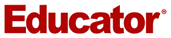Over 100+ comprehensive high school, college, and university courses taught by passionate educators.

## Searchable Lessons

All lessons are segmented into easily searchable and digestible parts. This is to save you time.

## Study Guides, Worksheets and Extra Example Lessons

Practice makes perfect!

## Start Learning Now

Our free lessons will get you started ( Adobe Flash ® required). Get immediate access to our entire library.

## Membership Overview

• *Ask questions and get answers from the community and our teachers!
• Practice questions with step-by-step solutions.
• Track your course viewing progress.
• Accessible anytime, anywhere with our Android and iOS apps.

## Free Printable trigonometry Worksheets for 10th Grade

Trigonometry-focused math worksheets for Grade 10 students, designed to help teachers and students discover essential concepts and skills. Download and print these free resources from Quizizz for an interactive learning experience.## Recommended Topics for you

• converse of pythagoras theorem
• pythagorean theorem
• trigonometric identities
• law of sines
• trigonometric ratios sin cos tan csc sec and cot
• inverse trigonometric functions
• trigonometric equations
• law of cosines
• graph sine functions10th - 12th## Explore trigonometry Worksheets by Grades

Explore trigonometry worksheets for grade 10 by topic, explore other subject worksheets for grade 10.

• social studies

## Explore printable trigonometry worksheets for 10th Grade

Trigonometry worksheets for Grade 10 are an essential resource for teachers looking to help their students master the fundamental concepts of trigonometry. These worksheets cover a wide range of topics, including angles, triangles, sine, cosine, and tangent functions, as well as their applications in real-world situations. By incorporating these worksheets into their lesson plans, teachers can provide their students with ample opportunities to practice and reinforce their understanding of trigonometry concepts. Furthermore, these worksheets can be easily adapted to suit the needs of individual students, making them an invaluable tool for differentiation in the classroom. In conclusion, trigonometry worksheets for Grade 10 are a must-have for any math teacher looking to enhance their students' learning experience.

Quizizz is an excellent platform for teachers to access a variety of resources, including trigonometry worksheets for Grade 10, math quizzes, and other engaging learning materials. This platform allows teachers to create interactive quizzes and games that can be used to supplement their existing lesson plans, providing students with a fun and engaging way to learn and practice new concepts. Additionally, Quizizz offers a wealth of pre-made quizzes and worksheets that cover a wide range of topics, making it easy for teachers to find and implement resources that align with their curriculum. Teachers can also track their students' progress and performance on these quizzes and worksheets, allowing them to identify areas where students may need additional support or practice. Overall, Quizizz is an invaluable tool for teachers looking to enhance their students' understanding of Grade 10 math concepts, including trigonometry.## Trigonometry (C) Word Problems worksheet

Total reviews: (0), trigonometry (c) word problems worksheet description.

This worksheet has identical questions to Trigonometry (C) Word Problems (With Clues) but without the added diagrams.

This set of 10 questions will give learners an opportunity to practise applying their knowledge of right-angled trigonometry (SOHCAHTOA).

The worded problems will involve drawing a diagram to fit with the scenario and then using sin, cos or tan to calculate a missing length or angle.

## Related to Trigonometry (C) Word Problems worksheet:

We love to get your feedback:.

Get 25% off Premium Membership with code BLACKFRIDAY until midnight Friday😀

## Get 20 FREE MATHS WORKSHEETS

Fill out the form below to get 20 FREE maths worksheets!## Word Problems Trigonometry Worksheet

Word Problems Trigonometry Worksheet – Word problems are an essential aspect of maths education. They require students to apply their math understanding to real-world situations, enhancing their problem solving skills and thinking capabilities. In this article, we’ll explore different types of word problems and how they can be solved, as well as provide ways to create engaging word problem worksheets.## Types of Word Problems:

• Word Problems with Addition: Addition word problems are problems that require one or two numbers in order to figure out the total. Example In the following example, if Sarah had 5 apples and the friend she is with gives her three more What is the number of apples she currently have?
• Subtraction Word Problems: Subtraction word problems require subtracting one number from another , in order to calculate the difference. Example For example: If there are 8 kids playing in a playground and 3 of them go home, how many are left at the playground?
• word problems:Multiplication word problems require adding two or more figures to identify the product. Example: If an example box contains six rows of 4 cupcakes How many cupcakes are inside the box?
• Division Word Problems: The division challenges involve splitting one number by another , in order to find the amount of quotient. Example for example: If you have 24 cupcakes in the kitchen and they need to be distributed evenly among six kids What is the number of cupcakes each child receive?
• Multi-Operation Word Problems: Mixed operation word problems involve various operations to arrive at an answer. Example A book that costs $10 and Mark does not have$25 how much change will he make in the event that he purchases the book , along with a pen costing \$5?## How to Solve Word Problems:

• Learn the issue Take the time to read the issue carefully and then identify what it’s trying to locate.
• Select the operation Find out the mathematical operation(s) are needed to complete the task.
• Select the right formula: Select the most appropriate formula or method of solving the issue.
• In order to solve the problem, you must use the formula, method or formula that you chose to solve the issue. Be sure to document your work and double-check the calculations.
• Answer the question: After solving the puzzle, go over the answer for accuracy and ensure that it’s logical within its context. You can also confirm your answer by using a different method.## Tips for Creating Word Problems Worksheets:

• Employ real-world scenarios. the word problems interesting and relatable by using real-world scenarios. For instance an issue with a word that is about baking cookies is far more interesting than any generic math problem.
• Increase the difficulty: Create word problems that vary in difficulty to challenge students of various skill levels. You can also incorporate multiple options to further increase amount of work.
• Multiple operations: Encourage the use of critical thinking by incorporating word problems that involve multiple tasks. This can also help students develop their problem-solving abilities.

## Conclusion:

Word problems play a significant aspect of mathematics education since they allow students to apply their mathematical knowledge to real-world situations. By creating exciting word problem worksheets and solving them using the steps provided in these, students can build their problem-solving abilities and develop skills of critical thinking.

## Gallery of Word Problems Trigonometry WorksheetSave my name, email, and website in this browser for the next time I comment.#### IMAGES

1. Trigonometry Word Problems Worksheet2. Trigonometry Word Problems Worksheet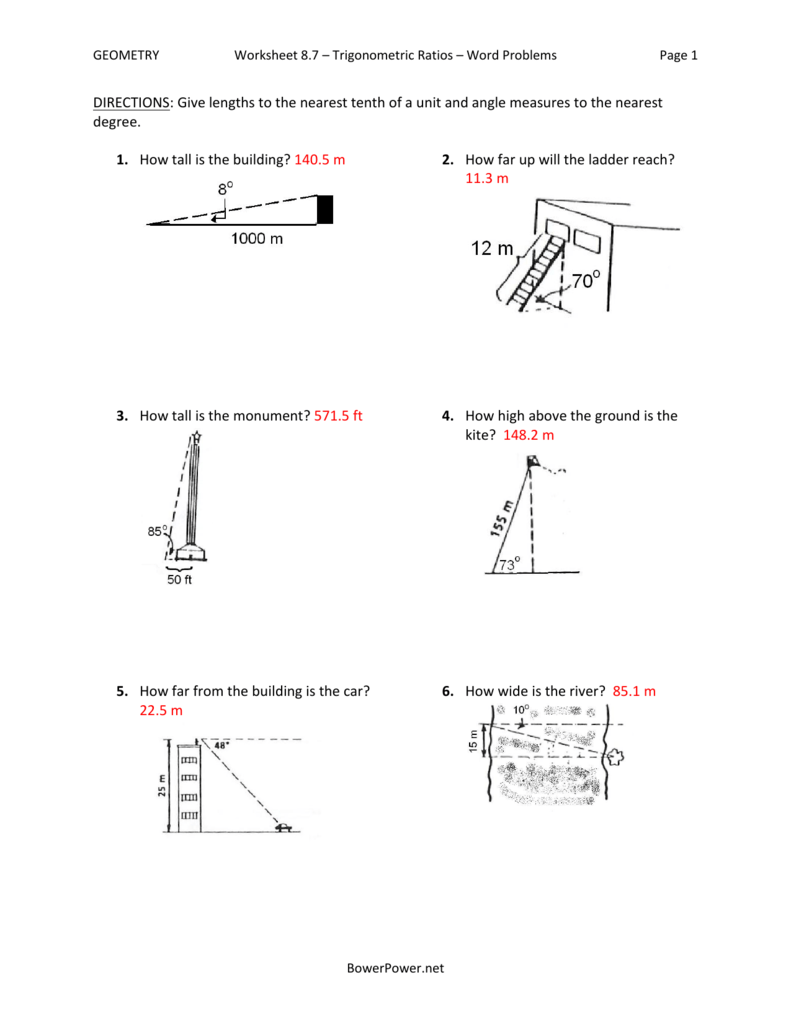3. Applied Trigonometry Problems Day 2 Worksheet for 9th4. Trigonometry Review Sheet5. Right Triangle Trigonometry Worksheet Luxury Trigonometry Word Problems6. 14 Trigonometry Trig Worksheets / worksheeto.com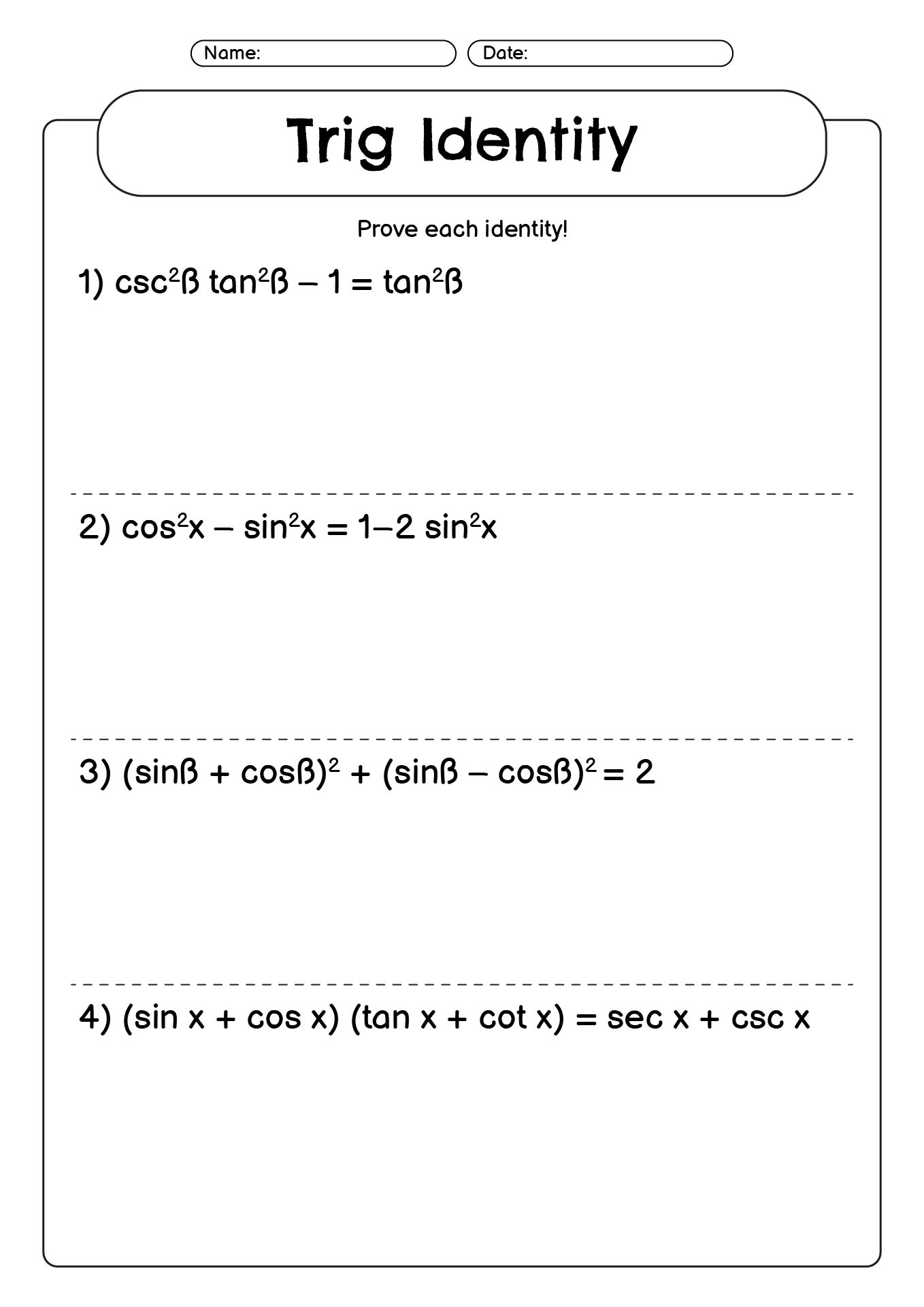#### VIDEO

1. IMPORTANT PROBLEMS TRIGONOMETRY NCERT CLASS 10TH BOARD EXAMINATION 2023_2024

2. P1

3. #trick trigonometry worksheet #**

4. TRIGONOMETRY IDENTITY PROBLEM 2

5. Applications of Trigonometry

6. TRIGONOMETRY RATIOS 0F COMPLEMENTARY ANGLE FOR CLASS 10th

1. TRIGONOMETRY WORD PROBLEMS WORKSHEET WITH ANSWERS

6. A ladder is leaning against a vertical wall makes an angle of 20° with the ground. The foot of the ladder is 3 m from the wall. Find the length of ladder. 7. A kite is flying at a height of 65 m attached to a string. If the inclination of the string with the ground is 31°, find the length of string. 8.

2. Trigonometry Word Problems Worksheets

Most worksheets contain between eight and eleven problems. When finished with this set of worksheets, students will be able to solve word problems that involve trigonometry. These worksheets explain how to solve word problems by using trigonometry. Sample problems are solved and practice problems are provided.

3. Trigonometry Word Problems Practice

1. From a point on the ground 47 feet from the foot of a tree, the angle of elevation of the top of the tree is 35º. Find the height of the tree to the nearest foot. 2. An 8 foot metal guy wire is attached to a broken stop sign to secure its position until repairs can be made.

4. Trigonometric Word Problems Worksheets

Trigonometric Word Problems Worksheets. How to Solve Trigonometric Word Problems - Students often get to solving the Trigonometry Problems stage when they are in the ninth grade, and it can get tricky at times. In this topic, we will be covering a general or basic idea regarding Solving Trigonometry Problems along with some useful tips. ...

5. 4.1.7: Trigonometry Word Problems

One application of the trigonometric ratios is to find lengths that you cannot measure. Very frequently, angles of depression and elevation are used in these types of problems. Angle of Depression: The angle measured down from the horizon or a horizontal line. Figure 4.1.7. 1. Angle of Elevation: The angle measured up from the horizon or a ...

6. Trigonometry

There are two options: Option 1: find the angle inside the triangle that is adjacent (next door) to the angle of depression. This adjacent angle will always be the complement of the angle of depression, since the horizontal line and the vertical line are perpendicular (90º). In the diagram at the left, the adjacent angle is 52º.

7. Trigonometry Word Problems

Solution: We can find the height of the monument by using the tangent ratio and then adding the eye height of the student. tan 87.4 ∘ = h 25 h = 25 ⋅ tan 87.4 ∘ = 550.54. Adding 5 ft, the total height of the Washington Monument is 555.54 ft. According to Wikipedia, the actual height of the monument is 555.427 ft.

8. Solving Word Problems with Trigonometry

We are open Saturday and Sunday! Call Now to Set Up Tutoring: (888) 888-0446. » Solving Word Problems with Trigonometry. While waiting for your sister to finish her bungee jump, you decide to figure out how tall the platform she is jumping off is. You are standing feet from the base of the platform, and the angle of elevation from your ...

9. Trigonometry Worksheets

Each one has model problems worked out step by step, practice problems, as well as challenge questions at the sheets end. Plus each one comes with an answer key. (This sheet is a summative worksheet that focuses on deciding when to use the law of sines or cosines as well as on using both formulas to solve for a single triangle's side or angle)

10. Trigonometry Worksheets (Math Made Fun)

Explore the trigonometry worksheets in detail and come back each day to practice as much as you need to so you can feel comfortable performing all the steps involved in trigonometry problems. Trigonometry problems are not easy, but memorizing the functions and steps will help you to break down each problem and successfully solve them. Our ...

11. PDF Trigonometry Word Problems

Worksheet #1 Trigonometry Word Problems Trigonometry Exercises 1.From an observation tower near Lake Winnipeg, the angle of el-evation of a weather balloon is 68 . In the same planem 35 km away, the balloon is sighted from another location with an angle of elevation of 47 . Calculate the distance from the weather balloon

12. PDF Right Triangle Word Problems

1. Read the problem carefully. 2. Draw and label the triangle. 3. Set up the equation. 4. Solve the equation. 5. Write a therefore statement. Applications of Right Triangle Trigonometry Right Triangle Word Problems 1 A boy who is flying a kite lets out 300 feet of string which makes an angle of 60o with the ground.

13. PDF Right Triangle Trigonometry: Solving Word Problems

Applications of Trigonometry Solve each problem. Round to the nearest hundredth. 1.) A tower casts a shadow that is 60 feet long when the angle of elevation of the sun is 65˚. How tall is the tower? 2.) Matt is standing on top of a cliff 305 feet above a lake. The measurement of the angle of depression to a boat on the lake is 42˚.

14. Right triangle trigonometry word problems

Right triangle trigonometry word problems. Bugs Bunny was 33 meters below ground, digging his way toward Pismo Beach, when he realized he wanted to be above ground. He turned and dug through the dirt diagonally for 80 meters until he was above ground. What is the angle of elevation, in degrees, of Bugs Bunny's climb?

15. Word problems: two triangles involved (practice)

Word problems: two triangles involved. Google Classroom. A flag is posted on the top of a castle. A knight on the ground standing 30 m away from the castle notices that the angles of elevation of the top of the castle and the top of the flag are 30 ° and 45 ° respectively.

16. Geometry Worksheets

This Trigonometry Worksheet will produce multi-step trigonometric problems. This worksheet is a great resource for the 5th Grade, 6th Grade, 7th Grade, and 8th Grade. These Trigonometry Worksheets allow you to select different variables to customize for your needs. These Geometry worksheets are randomly created and will never repeat.

17. Results for trig word problems

In this engaging worksheet, the trig functions sine, cosine and tangent are reinforced as students discover the answer to the riddle.There are 8 questions including 2 word problems.This product can be used forskill practicegroup workindividual workhomeworkreviewAnd more!This product is part of a bundle! Click here to find more activities like ...

18. 50. [Word Problems and Applications of Trigonometry]

Time-saving lesson video on Word Problems and Applications of Trigonometry with clear explanations and tons of step-by-step examples. Start learning today! ... Word Problems and Applications of Trigonometry. Slide Duration: Table of Contents. Section 1: Introduction. Introduction to Precalculus. 10m 3s. Intro. 0:00. Title of the Course.

19. Trigonometry Worksheets

Trigonometry Worksheet Answer Page. Now you are ready to create your Trigonometry Worksheet by pressing the Create Button. If You Experience Display Problems with Your Math Worksheet. This Trigonometry Worksheet will produce multi-step trigonometric problems. This worksheet is a great resource for the 5th Grade, 6th Grade, 7th Grade, and 8th Grade.

20. Free Printable trigonometry Worksheets for 10th Grade

Explore trigonometry Worksheets for grade 10 by Topic. converse of pythagoras theorem. pythagorean theorem. trigonometric identities. law of sines. trigonometric ratios sin cos tan csc sec and cot. inverse trigonometric functions. radians and degrees. radians and arc length.

21. Trigonometry (C) Word Problems Worksheet

This worksheet has identical questions to Trigonometry (C) Word Problems (With Clues) but without the added diagrams. This set of 10 questions will give learners an opportunity to practise applying their knowledge of right-angled trigonometry (SOHCAHTOA). The worded problems will involve drawing a diagram to fit with the scenario and then using ...

22. Word Problems Trigonometry Worksheets

Displaying all worksheets related to - Word Problems Trigonometry. Worksheets are Trigonometry word problems, Right triangle trigonometry word problems, Applications of right triangles and trig functions, Trigonometry work t1 labelling triangles, Ac unit 1 work 11 name steps to solving, Trigonometry packet geometry honors, Grade 11 general mathematics trigonometry, Periodic trig function models.

23. Word Problems Trigonometry Worksheet

Word Problems Trigonometry Worksheet - Word problems are an essential aspect of maths education. They require students to apply their math understanding to real-world situations, enhancing their problem solving skills and thinking capabilities.

24. Exponential Functions Word Problems Worksheet

2013-12-02 Spectrum(R) Word Problems for grade 7 includes practice for essential math skills, such as real world applications, multi-step word problems, variables, ratio and proportion, perimeter, area and volume, percents, statistics and more. Spectrum(R) Word Problems supplement to classroom work and proficiency test preparation. The series ...